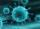# Sequence - 5 members

Write first five members of the sequenceResult

a1 =  6
a2 =  7
a3 =  12
a4 =  13
a5 =  18

#### Solution:Leave us a comment of example and its solution (i.e. if it is still somewhat unclear...):

Showing 0 comments:Be the first to comment!## Next similar examples:

1. Five membersWrite first 5 members geometric sequence and determine whether it is increasing or decreasing: a1 = 3 q = -2
2. Geometric sequence 4It is given geometric sequence a3 = 7 and a12 = 3. Calculate s23 (= sum of the first 23 members of the sequence).
3. GP membersThe geometric sequence has 10 members. The last two members are 2 and -1. Which member is -1/16?
4. SequenceFind the common ratio of the sequence -3, -1.5, -0.75, -0.375, -0.1875. Ratio write as decimal number rounded to tenth.
5. Six termsFind the first six terms of the sequence a1 = -3, an = 2 * an-1
6. Tenth memberCalculate the tenth member of geometric sequence when given: a1=1/2 and q=2
7. Geometric progression 2There is geometric sequence with a1=5.7 and quotient q=-2.5. Calculate a17.
8. GP - 8 itemsDetermine the first eight members of a geometric progression if a9=512, q=2
9. A perineumA perineum string is 10% shorter than its original string. The first string is 24, what is the 9th string or term?
10. BankPaul put 10000 in the bank for 6 years. Calculate how much you will have in the bank if he not pick earned interest or change deposit conditions. The annual interest rate is 3.5%, and the tax on interest is 10%.
11. PopulationWhat is the population of the city with 3% annual growth, if in 10 years the city will have 60,000 residents?
12. LogarithmDetermine the number whose decimal logarithm is -3.8.
13. VirusWe have a virus that lives one hour. Every half hour produce two child viruses. What will be the living population of the virus after 3.5 hours?
14. Quadratic function 2Which of the points belong function f:y= 2x2- 3x + 1 : A(-2, 15) B (3,10) C (1,4)
15. AsymptoteWhat is the vertical asymptote of ?
16. Theorem proveWe want to prove the sentense: If the natural number n is divisible by six, then n is divisible by three. From what assumption we started?
17. Geometric progression 48,4√2,4,2√2